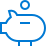SIP Calculator - Systematic Investment Plan Calculator Online

# SIP Calculator -Systematic Investment Plan Calculator OnlineInvest in small amounts regularlyOpt for monthly auto-paymentNo worries about market ups & downsPlan budget and expenses better
14 %
##### What is SIP Calculator?

SIP calculator is a simulation, which allows you to estimate the return on mutual fund investments made through SIP. Investing through SIPs in mutual funds is a popular investment option for millennials. SIP calculators are designed to give potential investors a heads up on their mutual fund investments.

However, the actual return from the mutual fund scheme varies depending on several factors. The SIP calculator does not account for the exit load and expense ratio (if any). It is an online tool to calculate the SIP amount to achieve your financial goals, based on an expected annual return.

##### How does SIP Calculator work?

A SIP plan calculator works by the values entered by the users.

You must enter the amount of investment, frequency of investment, duration of investment, and the expected returns. The SIP calculator is designed based on the compound interest formula. The compounded interest powers the mutual fund returns. ClearTax SIP Calculator shows the comparison of the returns offered by mutual funds with fixed deposits.

You can understand the workings of a SIP calculator with this formula.

FV = P [ (1+i)^n-1 ] * (1+i)/i
FV Future value or the amount you get at maturity.
PAmount you invest through SIP
iCompounded rate of return
nInvestment duration in months
rExpected rate of return
Take an example where you invest Rs 2,000 per month for a tenure of 24 months.
You expect a 12% annual rate of return (r).
You have i = r/100/12 or 0.01.
FV = 2000 * [(1+0.01) ^24 - 1] * (1+0.01)/0.01
You get Rs 54,486 at maturity.
##### How to Use the ClearTax Systematic Investment Plan Calculator?

Using the ClearTax systematic investment plan ( SIP )calculator is simple. To get an estimate of the return on mutual fund investments made through SIPs, you need to do the following:

• - Select the frequency of your SIP
• - Enter the amount you would like to invest in each SIP
• - Enter the duration of your SIP
• - Enter the expected rate of return

The SIP calculator displays the amount accumulated at the end of your SIP tenure. There is a comparison of the sum accumulated by your mutual fund investment with fixed deposits.